Helical Spring Calculators Enter value, select unit and click on calculate. Result will be displayed.

Calculate Spring Index
Calculate Total Shear Stress
Calculate Spring Axial Deflection
Calculate Spring Rate

 Web www.calculatoredge.com

Calculate Spring Index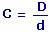C = Spring Index D = Spring Diameter d = Wire Diameter
 Enter your values: Spring Diameter (D): m Wire Diameter (d): m Result: Spring Index (C):

Calculate Total Shear Stress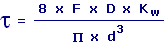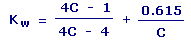t = Total Shear Stress F = Axial Force D = Spring Diameter d = Wire Diameter Kw = Wahl Factor C = Spring Index
 Enter your values: Axial Force (F): N Spring Diameter (D): m Wire Diameter (d): m Result: Total Shear Stress (t): Newton/Meter2 Spring Index (C): Wahl Factor (Kw):

Calculate Spring Axial Deflection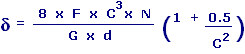d = Spring Axial Deflection F = Axial Force d = Wire Diameter N = Number Of Active Coils G = Modulus of Rigidity C = Spring Index
 Enter your values: Axial Force (F): N Spring Diameter (D): m Wire Diameter (d): m Number Of Active Coils (N): Modulus of Rigidity (G): N / m2 Result: Spring Axial Deflection (d):

Calculate Spring Rate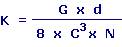K = Spring Rate d = Wire Diameter N = Number Of Active Coils G = Modulus of Rigidity C = Spring Index
 Enter your values: Spring Diameter (D): m Wire Diameter (d): m Modulus of Rigidity (G): N / m2 Number Of Active Coils (N): Result: Spring Rate (K):LINKSDISCLAIMERCONTACT US